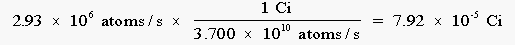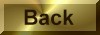Practice Problem 6

The most abundant isotope of uranium is 238U; 99.276% of the atoms in a sample of uranium are 238U.  Calculate the activity of the 238U in 1 L of a 1.00 M solution of the uranyl ion, UO22+.   Assume that the rate constant for the decay of this isotope is 4.87 x 10-18 disintegrations per second.

Solution

The rate at which the  238U isotope decays depends on the rate constant for this reaction (k) and the number of uranium atoms in the sample (N).   The rate constant for the decay of this nuclide was given in the statement of the problem and we can calculate the number of uranium atoms in the sample by noting that a liter of 1.00 M  UO22+ solution contains 1 mole of uranium atoms:

Rate = kN = (4.87 x 10-18 s-1)(6.02 x 1023 atoms) = 2.93 x 106 atom/s

To calculate the activity of this sample, we have to convert from disintegrations per second to curies:The curie is a very large unit of measurement.  Activities of samples handled in the laboratory are therefore often reported in millicuries (mCi) or microcuries (mCi).  This sample has an activity of 79.2 mCi.